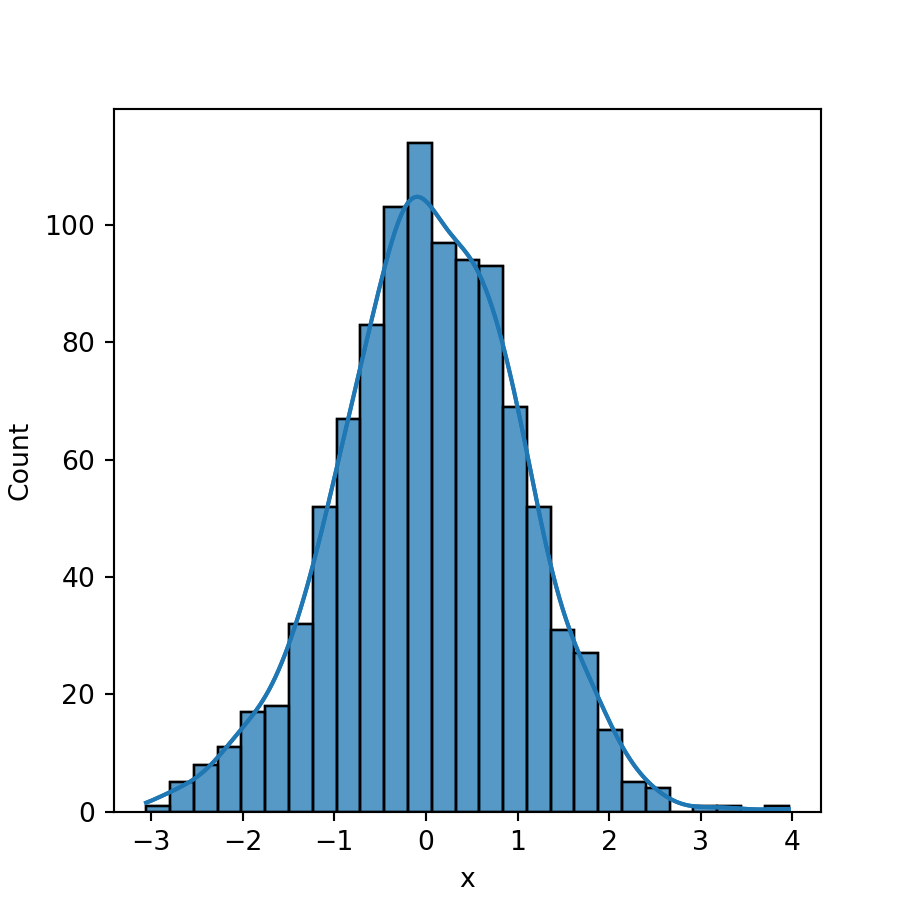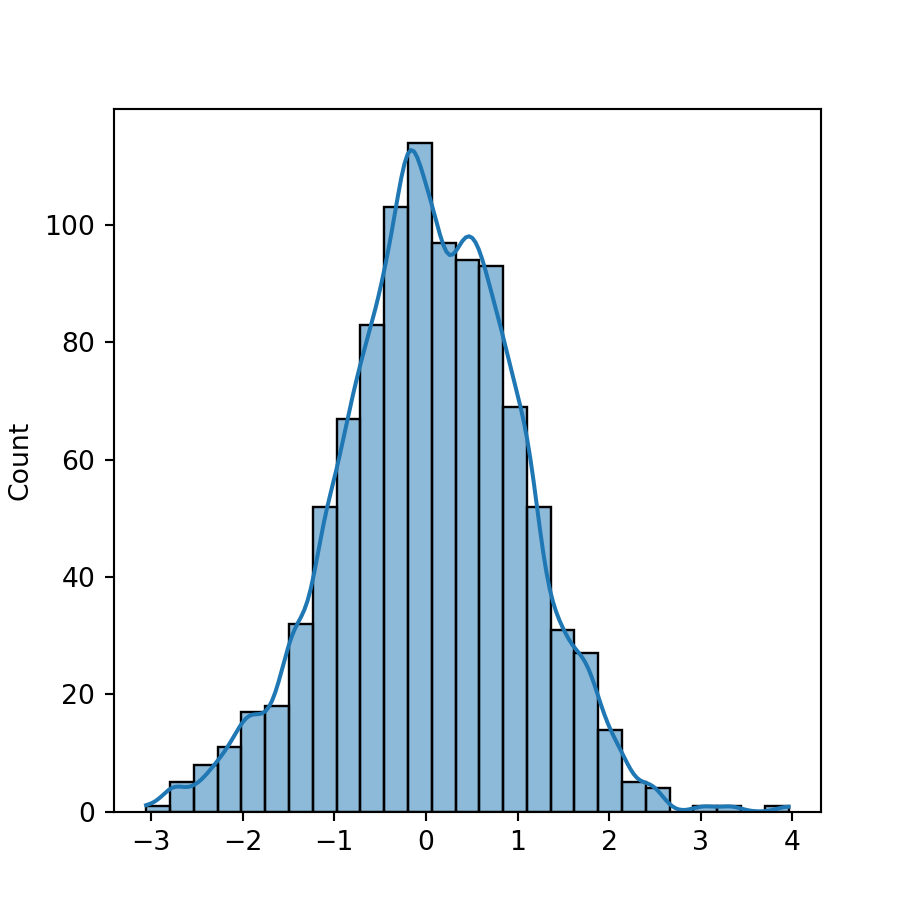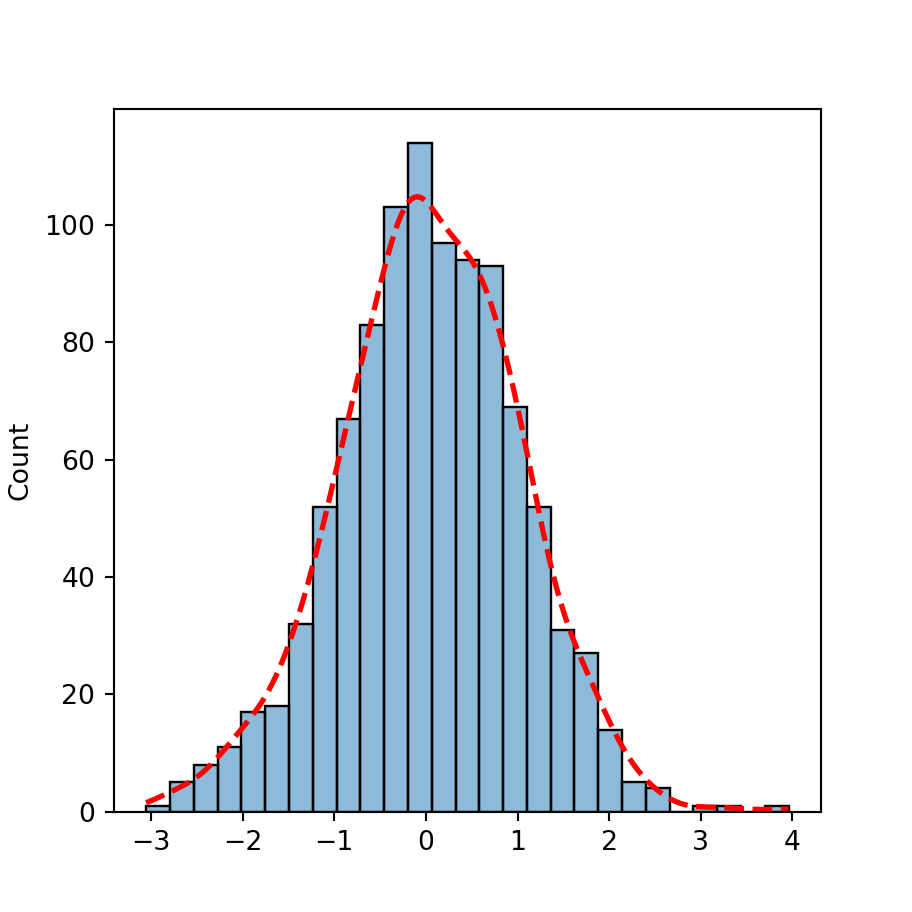# Histogram with density in seaborn

## Histogram with kernel density estimation

Setting the kde argument of the histplot function to True will add a curve representing the kernel density estimate of the data, calculated with the default parameters of the kdeplot function.

import numpy as np
import seaborn as sns

# Data simulation
rng = np.random.RandomState(1)
x = rng.normal(0, 1, size = 1000)
df = {'x': x}

# Histogram
sns.distplot(x = x, kde = True)

# Equivalent to:
sns.distplot(x = "x", kde = True, data = df)Kernel density estimate computation

Note that you can pass additional arguments to the kdeplot function with kde_kws. This will allow you to override the default arguments for the computation, such as the bandwidth used.

import numpy as np
import seaborn as sns

# Data simulation
rng = np.random.RandomState(1)
x = rng.normal(0, 1, size = 1000)
df = {'x': x}

# Histogram
sns.histplot(x = x, kde = True,
kde_kws = {'bw_adjust': 0.5})## Curve styling

The line_kws argument of the function can be used to customize the style of the kernel density estimate line passing a dictionary with the desired values. Note that you can override the default color of the line with set_color, as shown below.import numpy as np
import seaborn as sns

# Data simulation
rng = np.random.RandomState(1)
x = rng.normal(0, 1, size = 1000)
df = {'x': x}

# Histogram
sns.histplot(x = x, kde = True,
line_kws = {'linestyle':'dashed',
'linewidth':'2'}).lines.set_color('red')